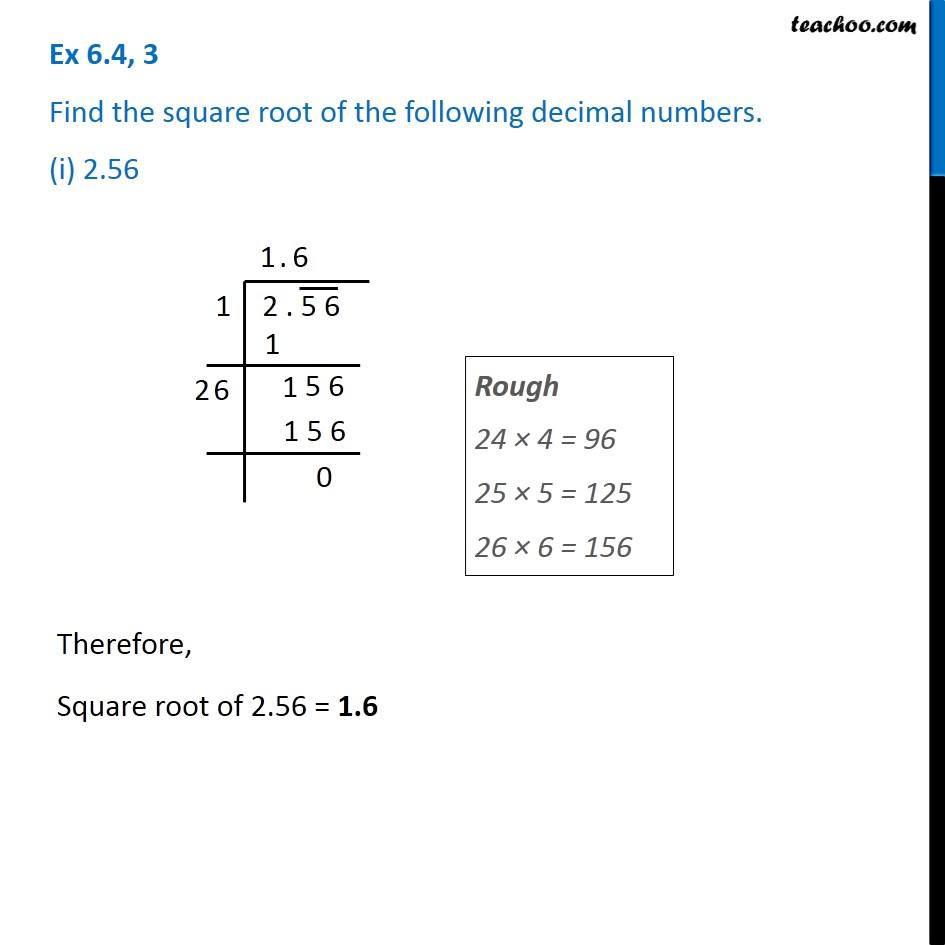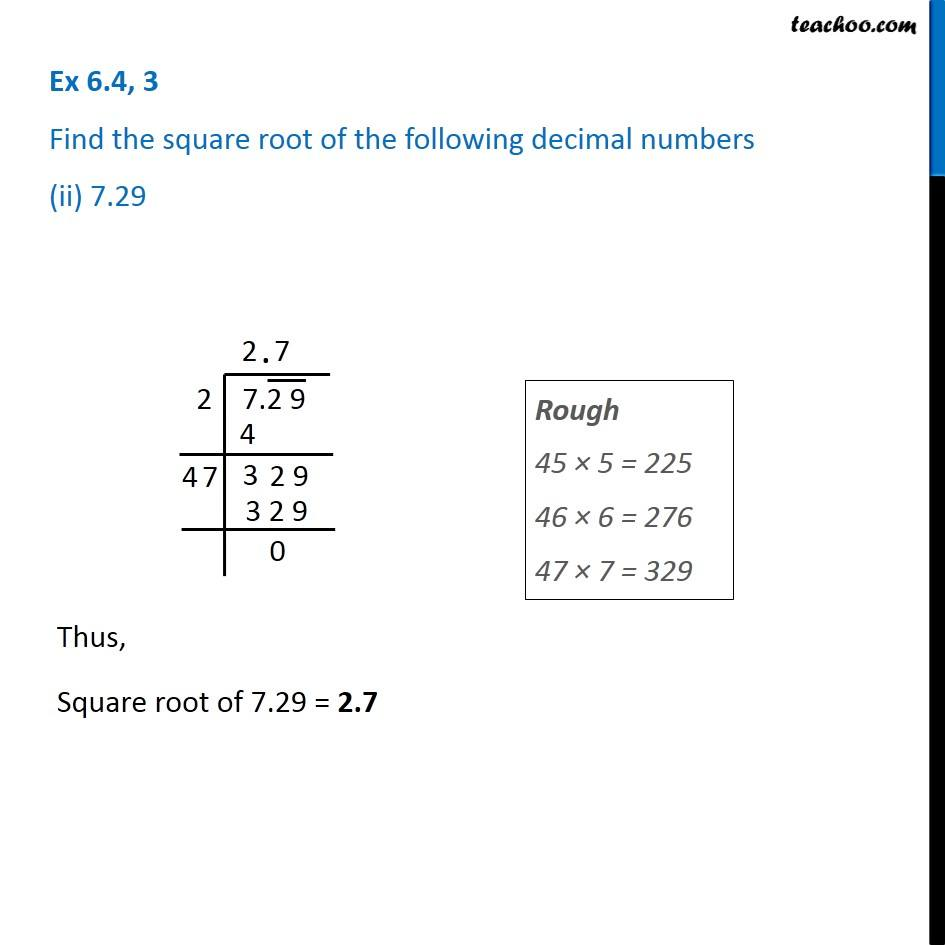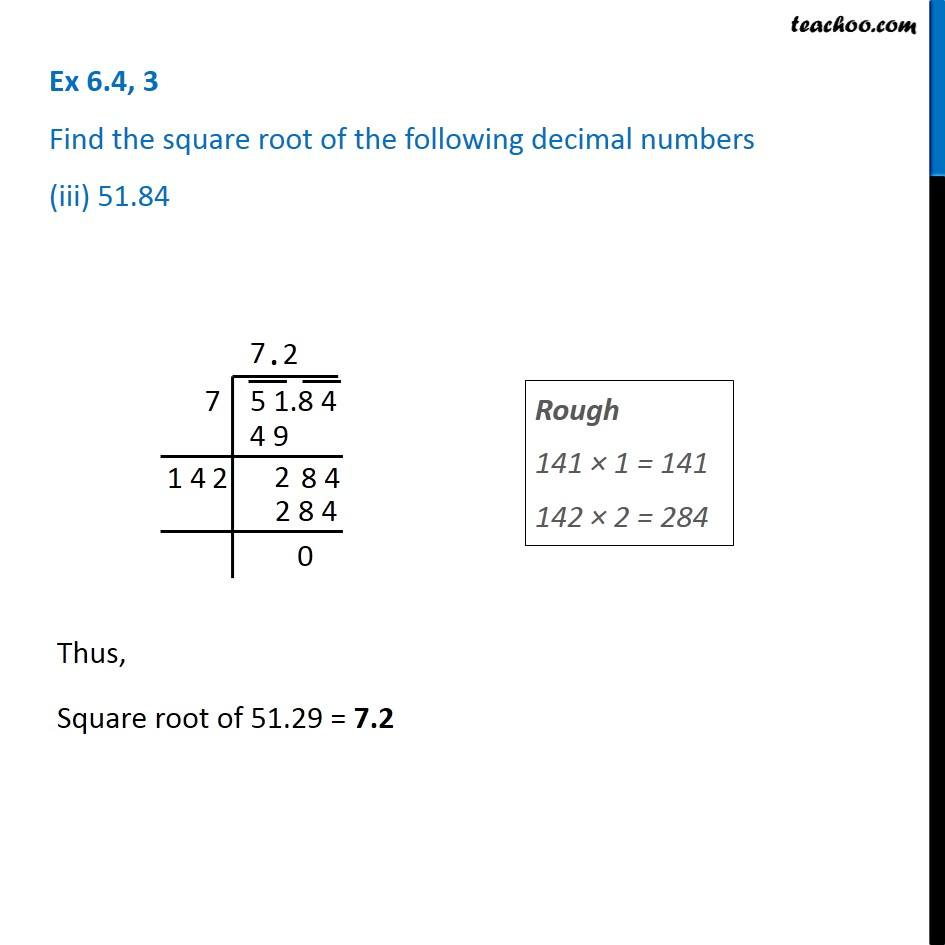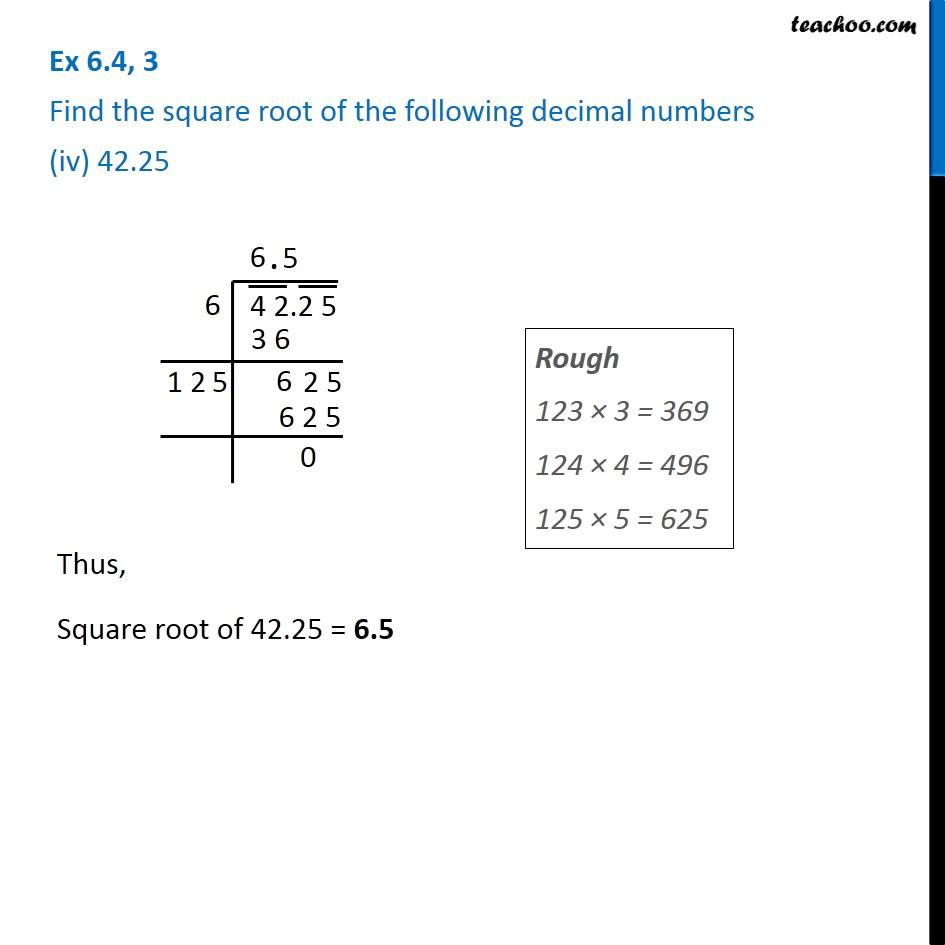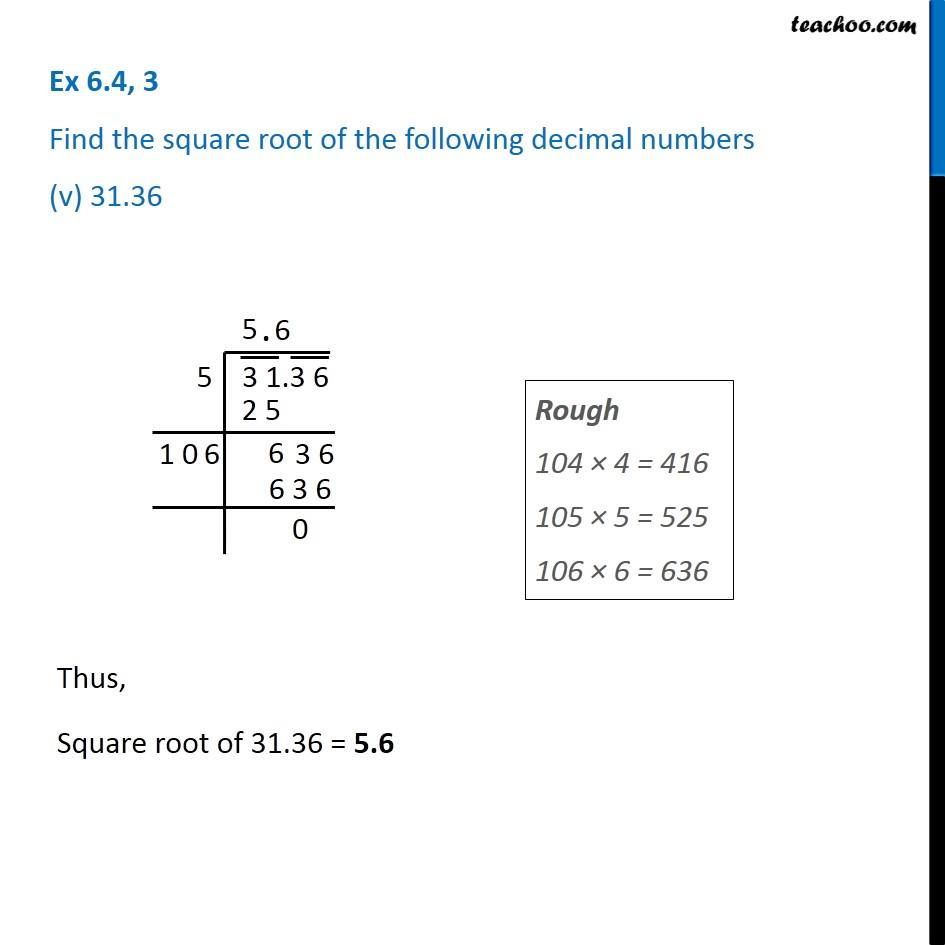1. Chapter 6 Class 8 Squares and Square Roots
2. Serial order wise
3. Ex 6.4

Transcript

Ex 6.4, 3 Find the square root of the following decimal numbers. (i) 2.56 Rough 24 × 4 = 96 25 × 5 = 125 26 × 6 = 156 Therefore, Square root of 2.56 = 1.6 Ex 6.4, 3 Find the square root of the following decimal numbers (ii) 7.29 Rough 45 × 5 = 225 46 × 6 = 276 47 × 7 = 329 Thus, Square root of 7.29 = 2.7 Ex 6.4, 3 Find the square root of the following decimal numbers (iii) 51.84 Rough 141 × 1 = 141 142 × 2 = 284 Thus, Square root of 51.29 = 7.2 Ex 6.4, 3 Find the square root of the following decimal numbers (iv) 42.25 Rough 123 × 3 = 369 124 × 4 = 496 125 × 5 = 625 Thus, Square root of 42.25 = 6.5 Ex 6.4, 3 Find the square root of the following decimal numbers (v) 31.36 Rough 104 × 4 = 416 105 × 5 = 525 106 × 6 = 636 Thus, Square root of 31.36 = 5.6

Ex 6.4